3. Transforming coordinates

Now that computerized data reductions of digital images (e.g., CCD frames) are commonplace, it is frequently necessary to transform positions of stars from one cartesian coordinate system to another. For most practical purposes we need only consider that the coordinate systems of two CCD frames (possibly from different telescopes) are related by a translation of the origin, a rotation, a scale change, and possibly a flip (for instance, if one frame is from a prime focus and the other is from a cassegrain focus), since the effects of higher-order distortions are usually negligible in a typical five-arcminute field of view. Therefore, our transformation equations can be written in the formSo, if we have n stars, we have 2n equations in four unknowns: A, B,, and. The chintzy way out is to solve the x and y equations separately:and then to fool around with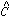,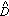,, and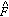to get some compromise average values forand. There's a more elegant way to do it, however.

First, let's rewrite our original equations a bit: letting C =cos() and D =sin(), we get(forgetting for the moment the possibility of a flip - I presume you'll know ahead of time when you've got one). These can be rewritten again,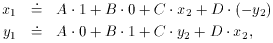and yet again,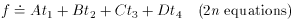where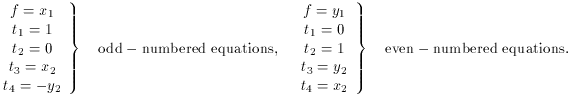Remember: I told you the tj, i can be anything you can assign a numerical value to. Now we can use vanilla least squares to get the answers we want: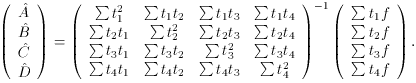If you really want to know them, you can now compute= sqrt(2 +2) and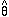= arctan(/) - no muss, no fuss, no compromises, just good, clean least squares straight from where you are to where you want to go.